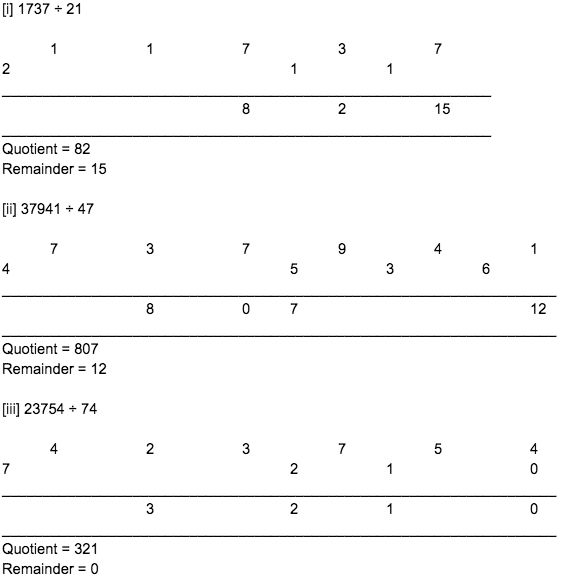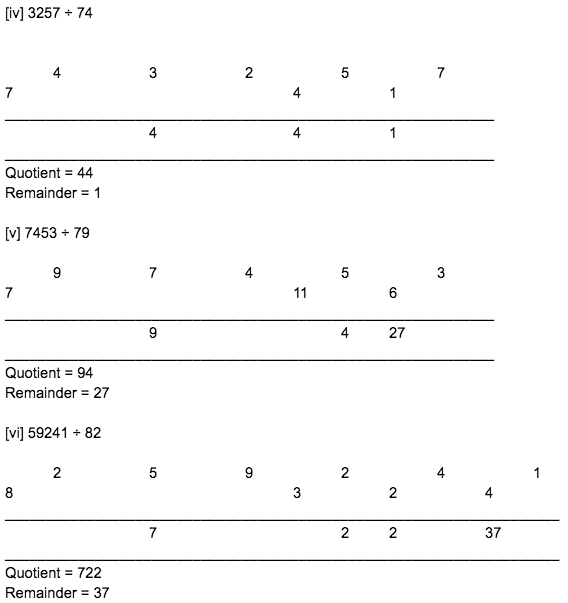# RBSE Maths Class 8 Chapter 5: Vedic Mathematics Important Questions and Solutions

RBSE Maths Chapter 5 – Vedic Mathematics Class 8 Important questions and solutions can be accessed here. The important questions and solutions of Chapter 5, available at BYJU’S, contain detailed step by step explanations. All these important questions are based on the new pattern prescribed by the RBSE. Students can also get the syllabus and textbooks on RBSE Class 8 solutions.

Chapter 5 of the RBSE Class 8 Maths will help the students to solve problems related to multiplication operation [Urdhwtirgbhyaam formula], multiplication by NIkhilam formula [sub-base], multiplication of three numbers, cube, Dhwajank method.

### RBSE Maths Chapter 5: Exercise 5 Textbook Important Questions and Solutions

Question 1: Multiply the following numbers using the Urdhwtirgbhyaam formula.

[i] 101 x 105

[ii] 11 x 15

[iii] 18 x 81

[iv] 121 x 129

Solution:

[i] 101 x 105

Here 101 is the multiplicand and 105 is the multiplier.

The number of groups in the case of three-digit numbers is 5. V, IV, III, II, I.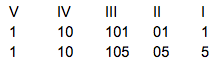Multiplication action and product

Multiplication action: 1 x 1 / 1 x 0 + 0 x 1 / 1 x 5 + 1 x 1 + 0 x 0 / 0 x 5 + 1 x 0 / 1 x 5

Product: 1 / 0 / 5 + 1 / 0 / 5

Addition: 1 / 0 / 6 / 0 / 5

101 x 105 = 10605

[ii] 11 x 15

Here 11 is the multiplicand and 15 is the multiplier.

The number of groups in the case of multiplication of two digits by two digits is 3. III, II, I.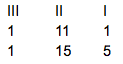Multiplication action and product

Multiplication action: 1 x 1 / 1 x 5 + 1 x 1 / 1 x 5

Product: 1 / 5 + 1 / 5

Addition: 1 / 6 / 5

11 x 15 = 165

[iii] 18 x 81

Here 18 is the multiplicand and 81 is the multiplier.

The number of groups in the case of multiplication of two digits by two digits is 3. III, II, I.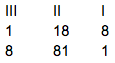Multiplication action and product

Multiplication action: 1 x 8 / 1 x 1 + 8 x 8 / 8 x 1

Product: 8 / 1 + 64 / 8

Addition: 8 / 65 / 8

8 + 6 / 5 / 8

14 / 5 / 8

18 x 81 = 1458

[iv] 121 x 129

Here 121 is the multiplicand and 129 is the multiplier.

The number of groups in the case of three-digit numbers is 5. V, IV, III, II, I.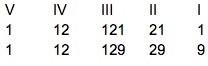Multiplication action and product

Multiplication action: 1 x 1 / 1 x 2 + 2 x 1 / 1 x 9 + 1 x 1 + 2 x 2 / 2 x 9 + 1 x 2 / 1 x 9

Product: 1 / 2 + 2 / 9 + 1 + 4 / 18 + 2 / 9

Addition: 1 / 4 / 14 / 20 / 9

1 / 4 + 1 / 4 + 2 / 0 / 9

1 / 5 / 6 / 0 / 9

121 x 129 = 15609

Question 2: Multiply the given numbers by Nikhilam formula.

[i] 48 x 51

[ii] 27 x 29

[iii] 36 x 34

[iv] 18 x 21

[v] 21 x 22 x 23

[vi] 31 x 28 x 27

[vii] 96 x 97 x 95

[viii] 18 x 18 x 18

[ix] 99 x 99 x 99

Solution:

[i] 48 x 51

Base = 10

Sub-base = 5 x 10 = 50

Sub-base digit = 50 ÷ 10 = 5

Deviation from sub-base = 48 – 50 = -2; 51 – 50 = 1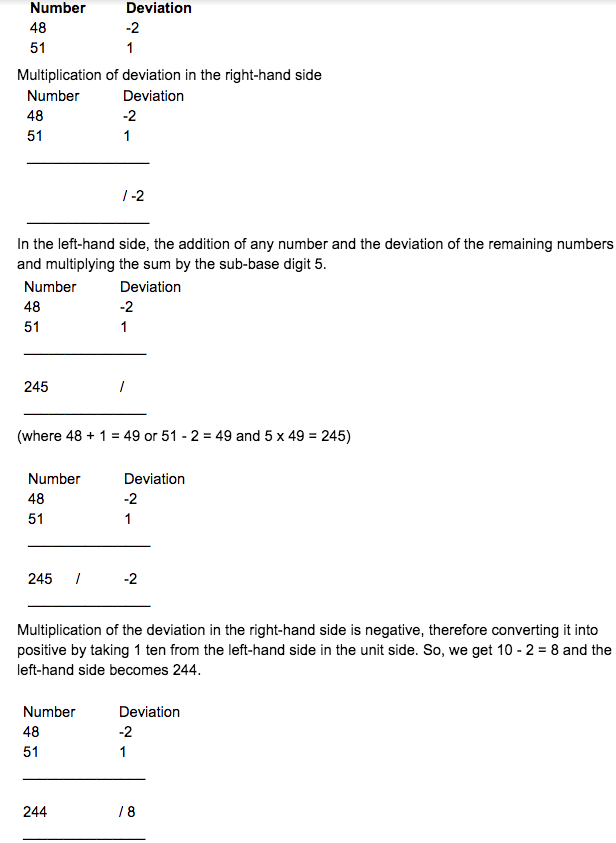= 2448

Thus, the product of 48 and 51 is 2448.

[ii] 27 x 29

Base = 10

Sub-base = 2 x 10 = 20

Sub-base digit = 20 ÷ 10 = 2

Deviation from sub-base = 27 – 20 = 7; 29 – 20 = 9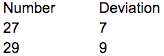Multiplication of deviation in the right-hand side.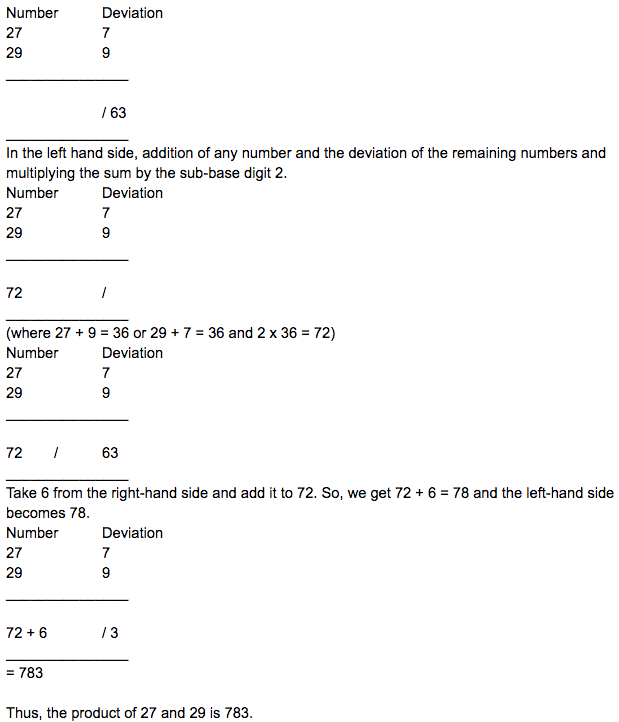[iii] 36 x 34

Base = 10

Sub-base = 3 x 10 = 30

Sub-base digit = 30 ÷ 10 = 3

Deviation from sub-base = 36 – 30 = 6; 34 – 30 = 4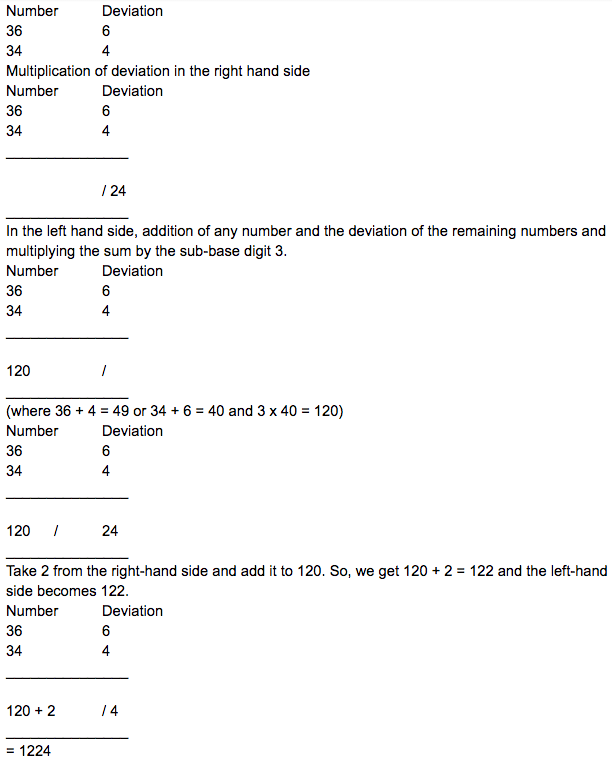Thus, the product of 36 and 34 is 1224.

[iv] 18 x 21

Base = 10

Sub-base = 2 x 10 = 20

Sub-base digit = 20 ÷ 10 = 2

Deviation from sub-base = 18 – 20 = -2; 21 – 20 = 1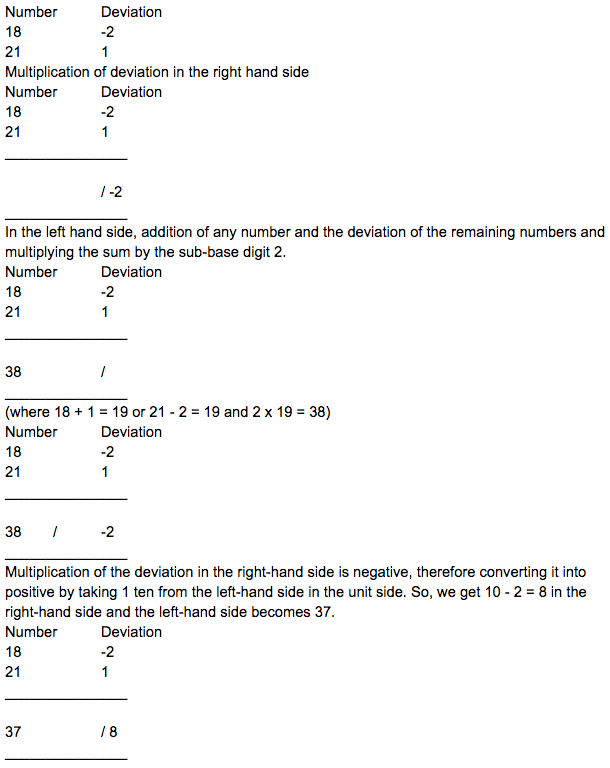= 378

Thus, the product of 18 and 21 is 378.

[v] 21 x 22 x 23

Base = 20

Deviation from the sub-base = +1, +2, +3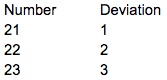Multiplication of deviation in the right-hand side: Product of the two deviations and their additions are made.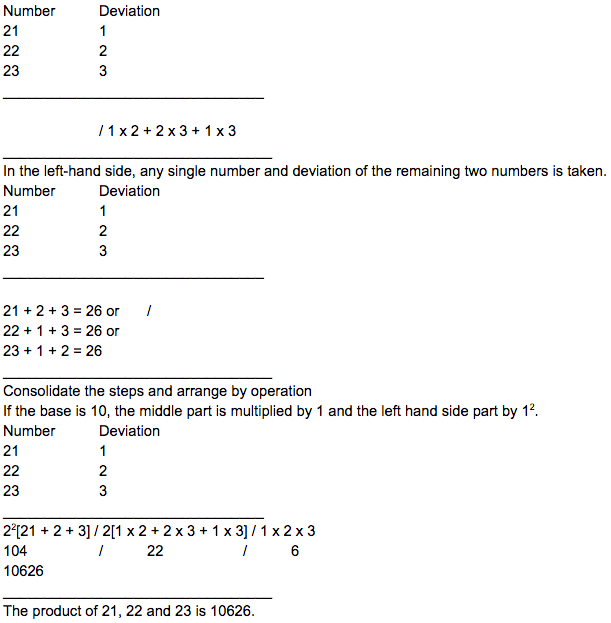[vi] 31 x 28 x 27

Base = 30

Deviation from the sub-base = +1, -2, -3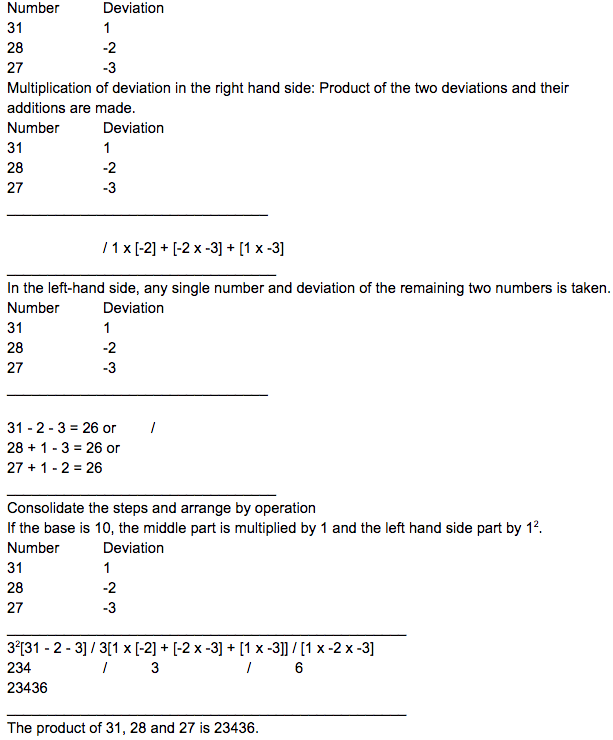[vii] 96 x 97 x 95

Base = 90

Deviation from the sub-base = +6, +7, +5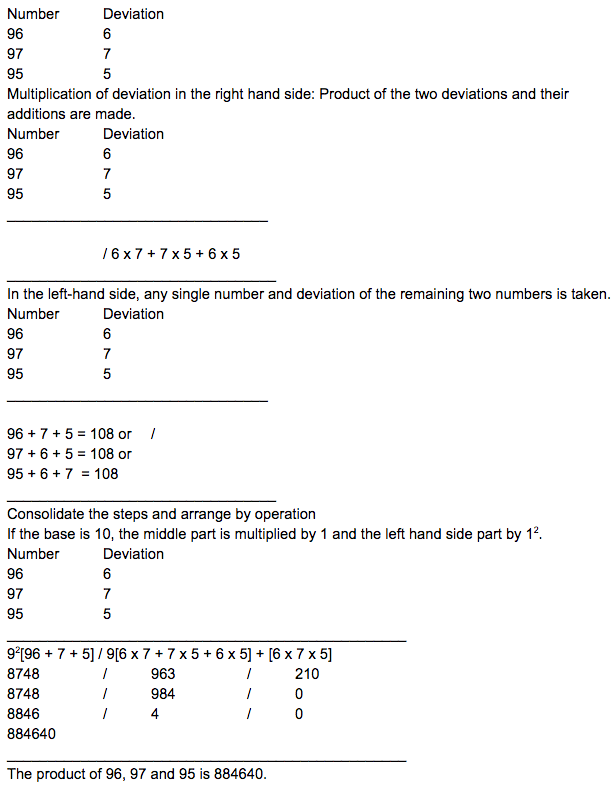[viii] 18 x 18 x 18

Base = 20

Deviation from the sub-base = -2, -2, -2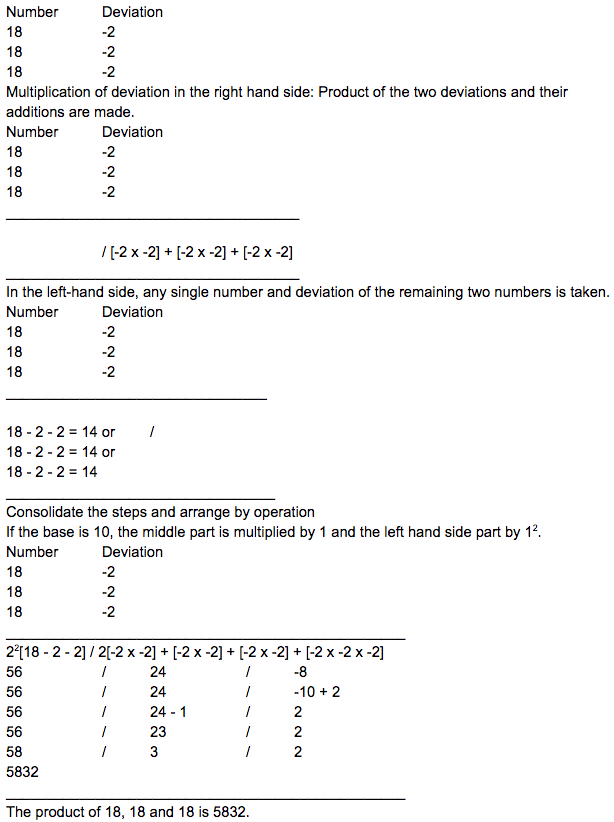[ix] 99 x 99 x 99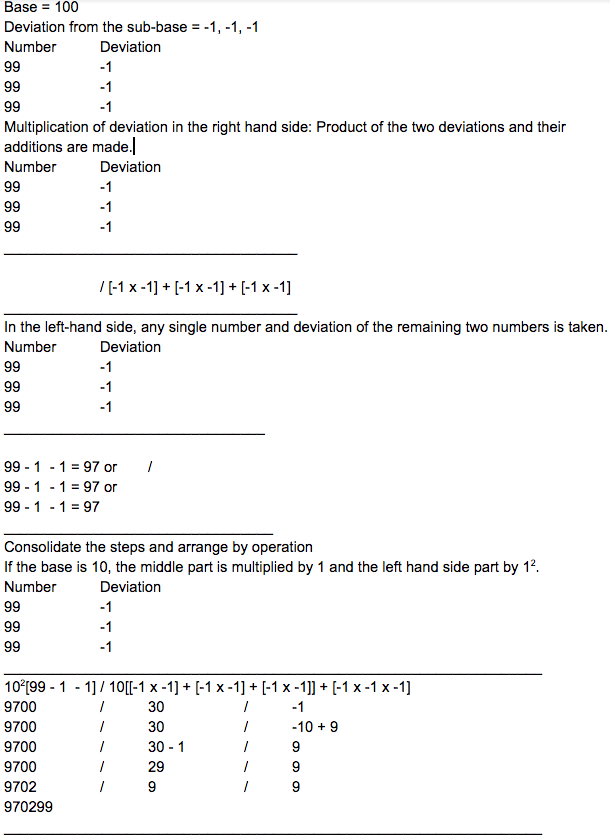The product of 99, 99 and 99 is 970299.

Question 3: Divide the following numbers using Dhwajank formula.

[i] 3987 ÷ 28

[ii] 5786 ÷ 78

[iii] 7396 ÷ 82

Solution: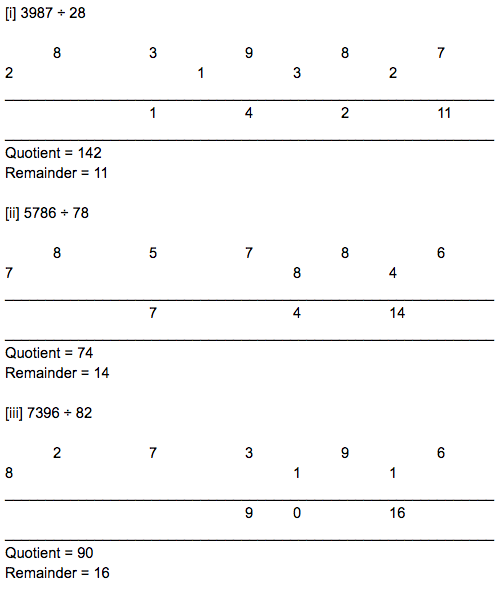### RBSE Maths Chapter 5: Additional Questions and Solutions

Question 1: Multiply the following numbers using the Urdhwtirgbhyaam formula.

[i] 15 x 12

[ii] 60 x 18

[iii] 71 x 8

[iv] 122 x 4

[v] 706 x 56

[vi] 497 x 173

Solution: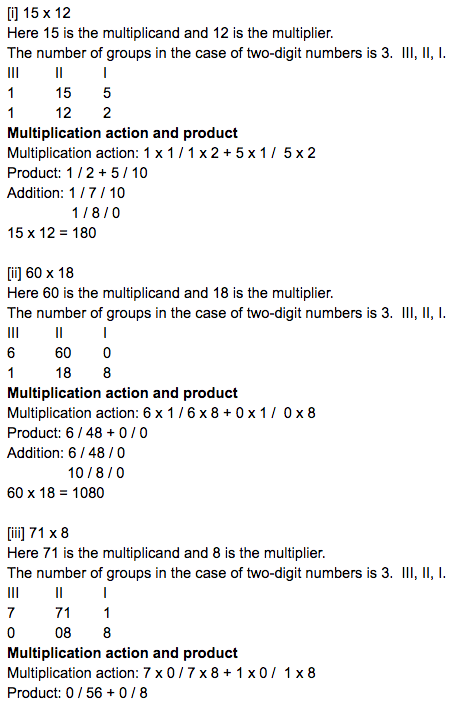Addition: 0 / 56 / 8

71 x 8 = 568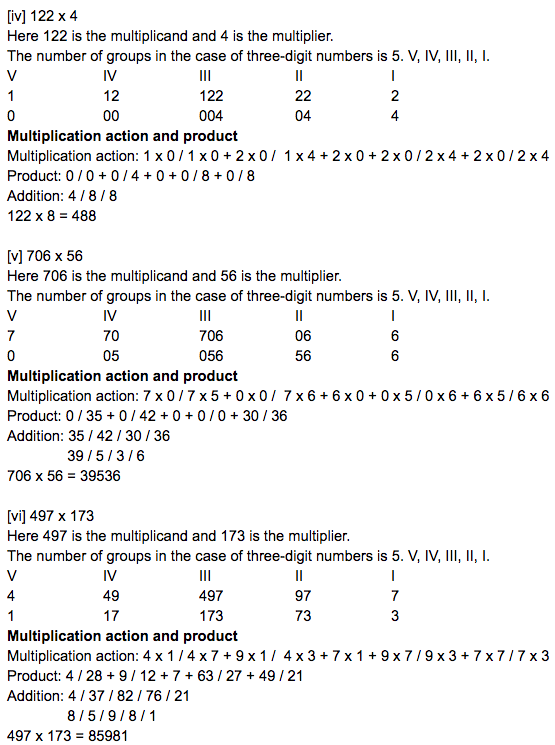Question 2: Find the product of the following by Nikhilam formula

[i] 11 x 15 [ii] 12 x 18 [iii] 19 x 17

[iv] 28 x 22 [v] 51 x 49 [vi] 99 x 96

Solution:

[i] 11 x 15

Base = 10

Sub-base = 1 x 10 = 10

Sub-base digit = 10 ÷ 10 = 1

Deviation from sub-base = 11 – 10 = 1; 15 – 10 = 5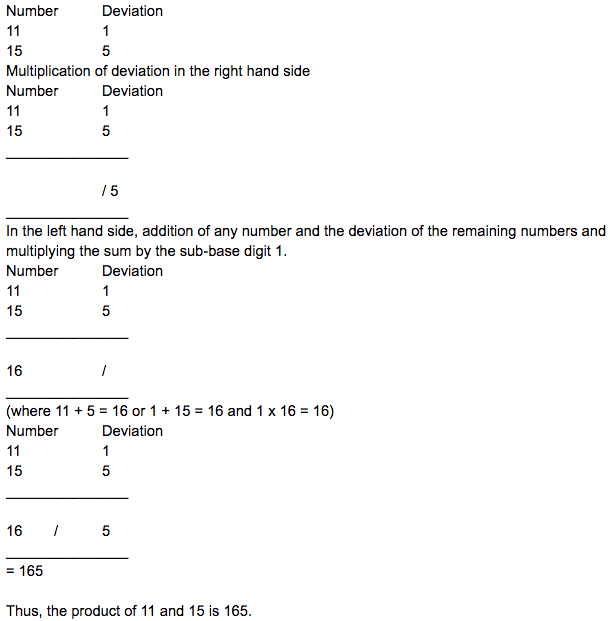[ii] 12 x 18

Base = 10

Sub-base = 1 x 10 = 10

Sub-base digit = 10 ÷ 10 = 1

Deviation from sub-base = 12 – 10 = 2; 18 – 10 = 8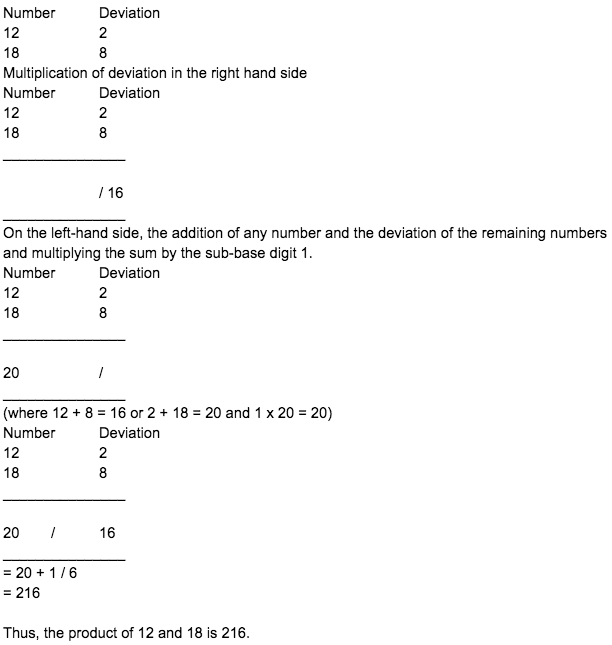[iii] 19 x 17

Base = 10

Sub-base = 1 x 10 = 10

Sub-base digit = 10 ÷ 10 = 1

Deviation from sub-base = 19 – 10 = 9; 17 – 10 = 7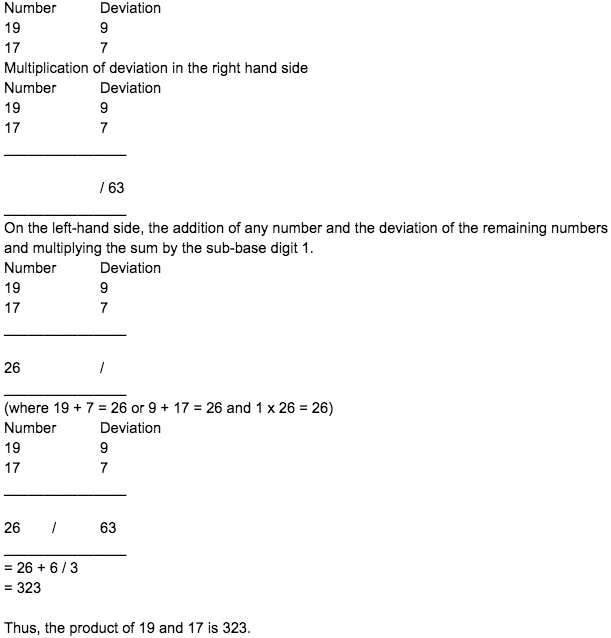[iv] 28 x 22

Base = 10

Sub-base = 2 x 10 = 20

Sub-base digit = 20 ÷ 10 = 2

Deviation from sub-base = 28 – 20 = 8; 22 – 20 = 2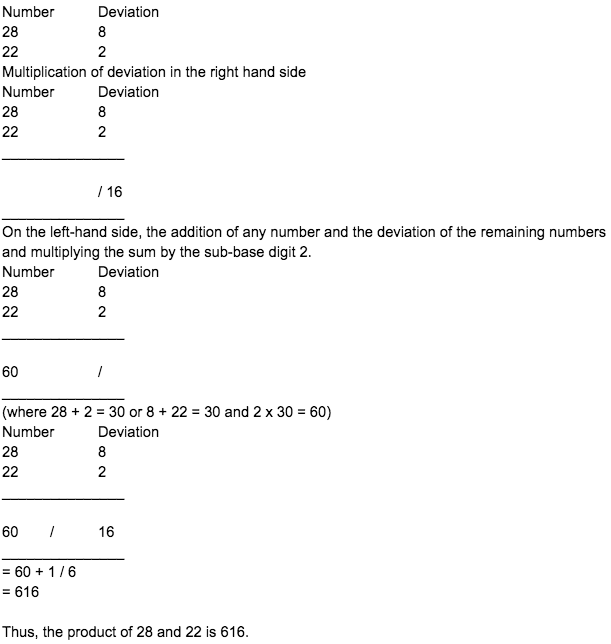[v] 51 x 49

Base = 10

Sub-base = 5 x 10 = 50

Sub-base digit = 50 ÷ 10 = 5

Deviation from sub-base = 51 – 50 = 1; 49 – 50 = -1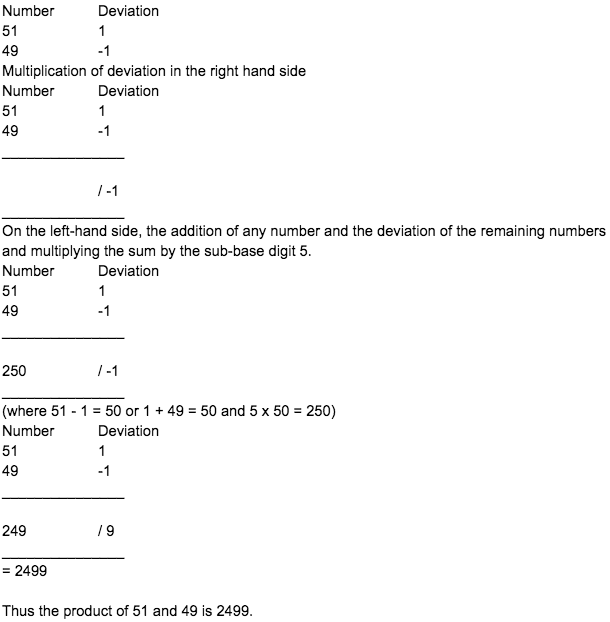[vi] 99 x 96

Base = 10

Sub-base = 9 x 10 = 90

Sub-base digit = 90 ÷ 10 = 9

Deviation from sub-base = 99 – 90 = 9; 96 – 90 = 6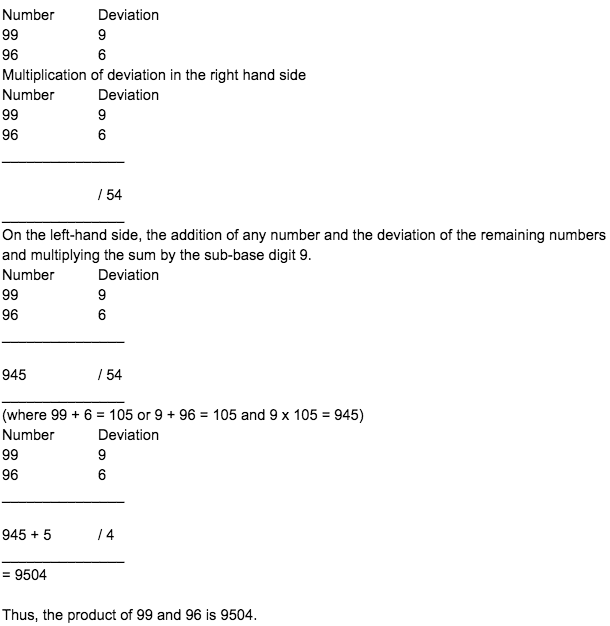Question 3: Multiply the following using the Nikhilam formula.

[i] 11 x 12 x 13

[ii] 8 x 9 x 10

[iii] 6 x 7 x 8

[iv] 27 x 28 x 29

[v] 98 x 99 x 99

[vi] 51 x 52 x 53

Solution:

[i] 11 x 12 x 13

Base = 10

Deviation from the sub-base = +1, +2, +3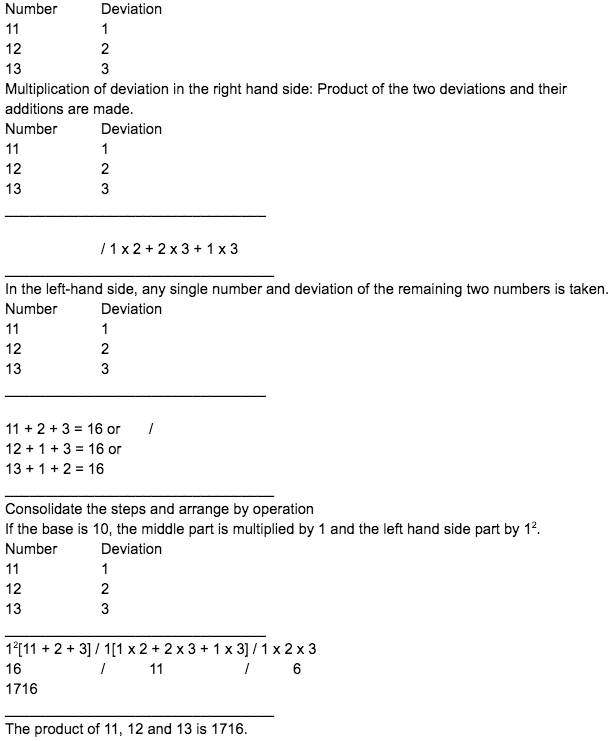[ii] 8 x 9 x 10

Base = 10

Deviation from the sub-base = -2, -1, 0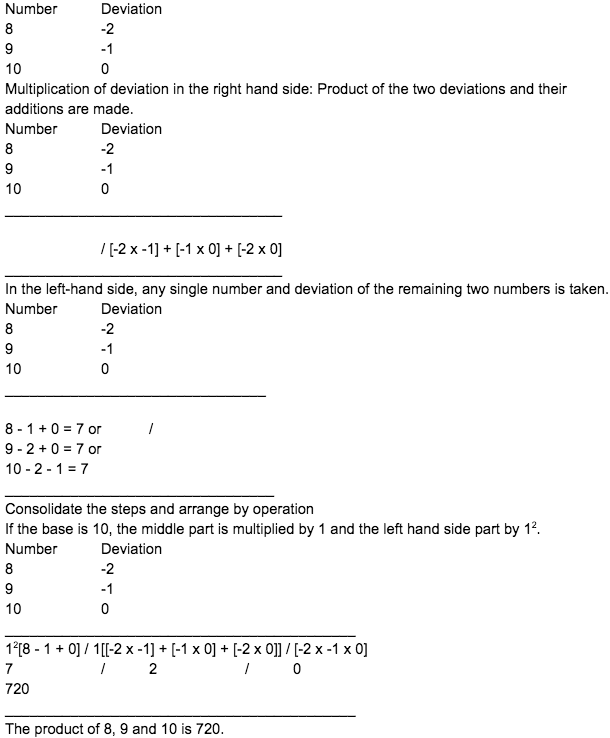[iii] 6 x 7 x 8

Base = 10

Deviation from the sub-base = -4, -3, -2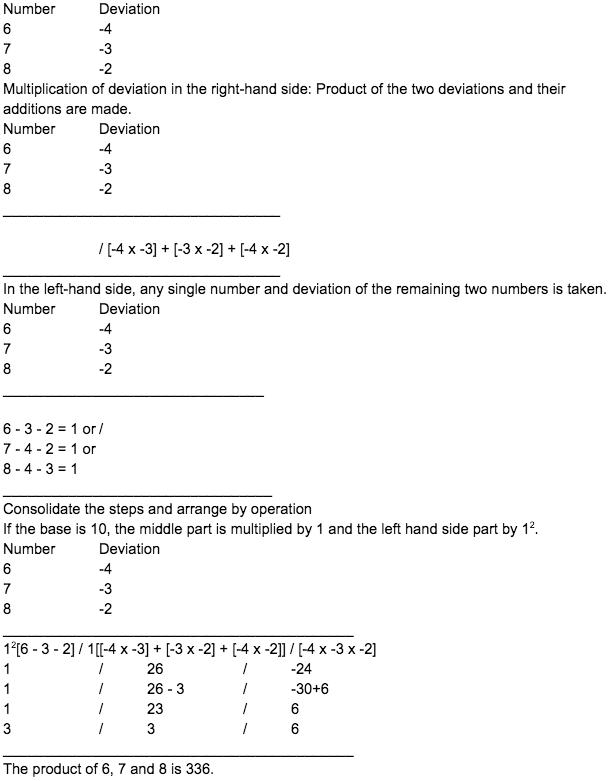[iv] 27 x 28 x 29

Base = 20

Deviation from the sub-base = 7, 8, 9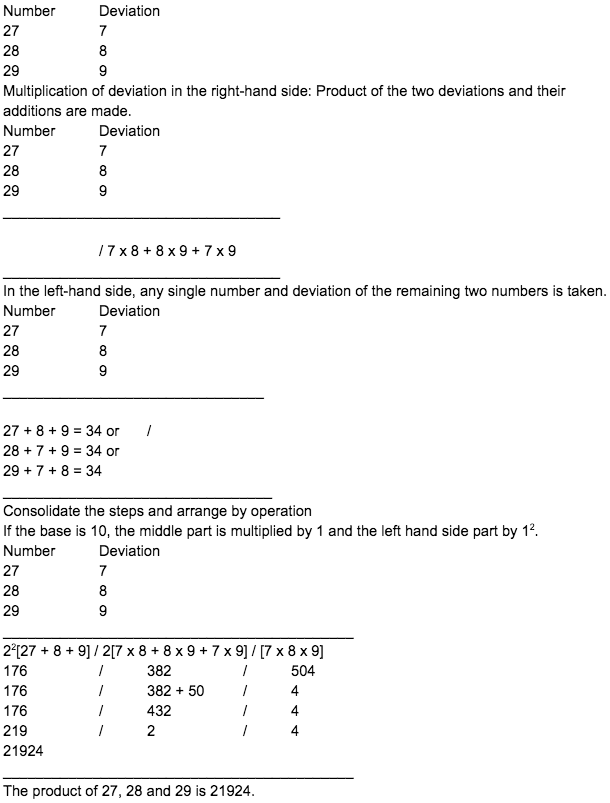[v] 98 x 99 x 99

Base = 90

Deviation from the sub-base = 8, 9, 9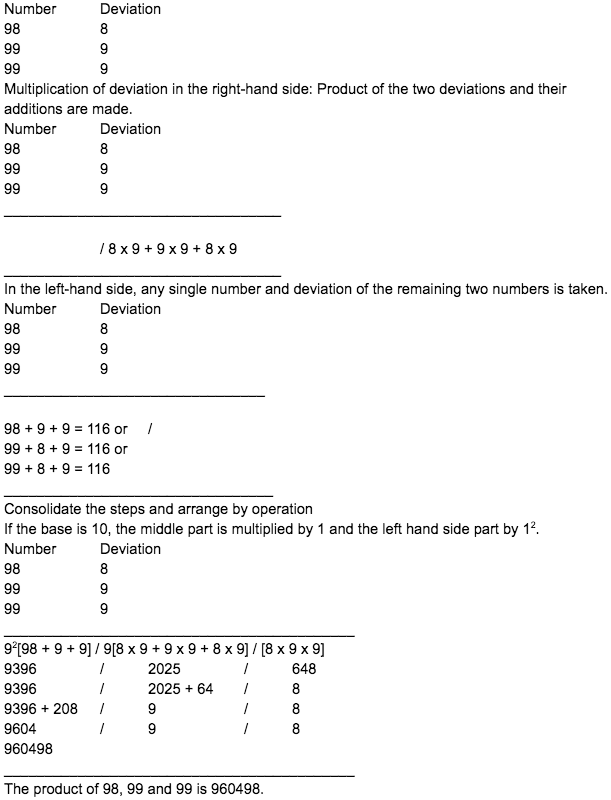[vi] 51 x 52 x 53

Base = 50

Deviation from the sub-base = 1, 2, 3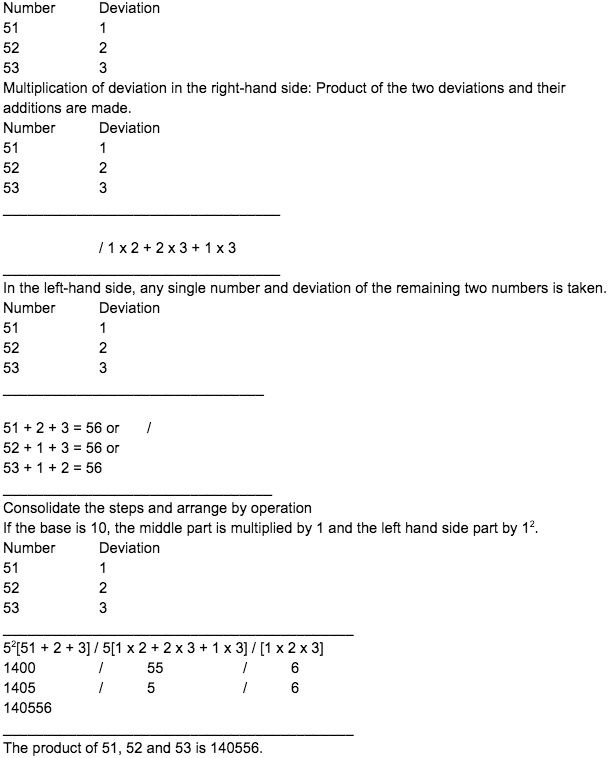Question 4: Find the quotient and remainder of the following numbers by Dhwajank formula.

[i] 1737 ÷ 21

[ii] 37941 ÷ 47

[iii] 23754 ÷ 74

[iv] 3257 ÷ 74

[v] 7453 ÷ 79

[vi] 59241 ÷ 82

Solution: